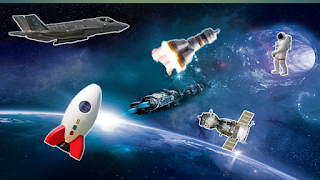### What is Physics? // Fundamental Units and Derived Units // System of Units

1. Introduction:
The branch of science which deals with the study of natural phenomena is called physics. To understand and know the physics we must be able to connect our theoretical description of nature with our experimental observations of nature. This connection can be made through quantitative measurements. In the field of physics, here we may deal with a large number of physical quantities like, length, mass, time, velocity. force, pressure etc. These quantities can provide clear understanding only if we will be able to measure them and express our conclusions into these measurements. To know the physical quantity quantitatively, measurement is required. Because of this reason, physics is sometimes called the science of measurement. Hence, the process of comparing an unknown physical quantity with a known fixed quantity is called the measurement. The quantity which is used as a standard of measurement is called the unit. Physical quantity is expressed quantitatively as the multiple of the unit taken.Figure: Space Science

Fundamental Units and Derived Units
Physical quantities can be divided into two groups- base quantities and derived quantities. The units for these quantities are called fundamental units and derived units.

Fundamental units:
Physical quantities which are independent and cannot be derived from other physical quantities are called fundamental quantities and so their units are called the fundamental or base units. The examples of fundamental physical quantities are mass, time, length, temperature, electric current, intensity of light and quantity of matter etc.

Derived units:
The units of physical quantities which can be expressed in terms of fundamental units are called derived units. Units of speed, force, momentum etc. are the examples of derived units.

Characteristics of a Standard Unit
Standard unit of physical quantity should have the following characteristics features:
i) It should be properly defined.
ii) It should remain constant.
iii) It should be easily available and reproducible.
iv) It should be universally accepted.
2. System of Units
Different systems of units are used in measurement. For example, MKS system, CGS system, FPS system. SI-units are mostly used in measurement in science and engineering.

MKS-system: According to this system, unit of length is meter, unit of mass is kilogram and unit of time is second.

CGS-system: In this system, length is measured in centimeter, mass in gram and time in second.

FPS-system: According to this system, length is measured in foot, mass in pound and time in second.

SI-units: In this system, seven basic and two supplementary units are considered. Length is taken in meter mass in kilogram, time in second, temperature in Kelvin, electric current in ampere, luminous intensity in candela and quantity of matter in mole. Two supplementary units are plane angle and solid angle, and their units are radian and steradian respectively.